• 3
•
•
•
•
•
•
•
3
Shares

# The frame of reference and pseudo force

In Physics what we do is observe Nature, different Phenomenon and describe our observations, i.e where the event happened when the event happened and this all depends on different observers.

For example: suppose a tree is 50 m far from you and someone is observing that tree behind you then for that observer the tree is 50+ meters far. so we know that these things depend on the observer.

To describe an observation(event) we need to
1. describe the location of the event. (x,y,z)
2. describe the time when the event happening.

so by specifying the above two, we describe the event as, chalk is at distance(x,y,z) w.r.t me at time t.
So a Frame of reference is something using which we specify an event.

A frame of reference consists of X, Y, Z mutually perpendicular axes. With origin as Observer
and something says a clock to measure time, all of these combined make a frame of reference, implies an observer with x, y, z axes, and a clock is a frame of reference.# Types Of Frame Of Reference.

In Physics We all know newton’s laws of Motion also 1st law. if you are a good observer (a physics student must be) you must be observed that in some cases newton’s first law appears to be invalid.

• Suppose we are sitting in running bus and we see that when the bus suddenly stopped all passengers experience jerk forward, but w.r.t us inside the bus we see there is no force acting on us, So as Per 1st Law as there is not any force acting on us we should not experience jerk at all, but in reality, we experienced.

• Another instance we are in a bus that is accelerating w.r.t ground implies it’s velocity is changing, now w.r.t. us from inside of bus we see the outside objects such as trees, etc accelerating to the opposite of motion of us, but there’s no net force on them but still they are accelerating. Here Newton’s first law is invalid w.r.t such an observer from inside accelerating bus.

• Also consider that we’re inside the bus moving with constant velocity i.e no acceleration, now w.r.t inside of bus we see each other passengers at rest and also no force acted on them so first law of Newton is valid there also if we observe the outside we see that different things like a tree, etc seems to be moving with constant velocity in the direction opposite to the motion of bus also since we see no net force on trees, etc w.r.t Newton’s first law is again valid as if there’s no net force and if the object is moving then it will continue to move with constant velocity.

Therefore We arrive at the point that there is some frame of reference (such as accelerating bus’s frame of reference) From which Newton’s First law does not hold good.

From the above discussion we concluded two types of frame of references:-

## Inertial Frame of Reference

frames of reference from which newton’s First Law Holds Good are known as inertial frames of reference or Galilean frame of reference or inertial space. by observing we say that all non-accelerating frames which are at rest or moving with constant velocity w.r.t each other are inertial frames.

## Non-Inertial Frame of Reference

A frame of reference from which newton’s first law does not valid (not holds good), all accelerating frames of reference are non-inertial frames.

# Pseudo force

## Introduction

So in a non-inertial frame, Newton’s law can’t be applied but we know that Newton’s laws are very useful it governs kinematics and dynamics of a body. Therefore to apply Newton’s laws from a non-inertial frame a method is developed in which while writing all the forces on a body an imaginary (pseudo) force is also added then Newton’s laws can be written and the correct velocity acceleration force etc parameters will be obtained. We are going to derive the whole using basic mathematics.

## Derivation

Consider a frame of reference S which is an inertial frame of reference and there is a frame of reference S’ which moves with constant acceleration “a” w r.t S and there is a particle “p” of mass m as shown in the figure.

Acceleration of p w.r.t frame s is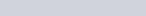Similarly acceleration of p w.r.t s’ is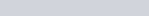As s’ is moving w.r.t s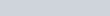Multiply both sides by m, then we get-

(1)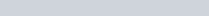Now from the above expression, we can see that force on particle p w.r.t S’ frame is coming to be different from the force on particle p w.r.t S frame.

But since we know that in non-inertial frames even if force is zero objects still got accelerating, therefore, the correct force and acceleration will be obtained as per Newton’s laws from inertial frames, therefore, to apply Newton’s law from non-inertial frame S’ the force on the body should be same as we get through the inertial frame.

We can see if we add an extra term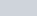to equation (1) , the force from the non-inertial frame will equal that from an inertial frame.
This extra term added is known as pseudo force as no such force acts in real it’s added to mathematically apply Newton’s law from non-inertial frames.

Now, we can mathematically apply Newton’s law to obtain velocity acceleration force, etc. For applying newton’s first law from non-inertial frames, we apply an additional Pseudo Force to object under study from non-inertial frames.

Stay tuned with Laws Of Nature for more useful and interesting content.